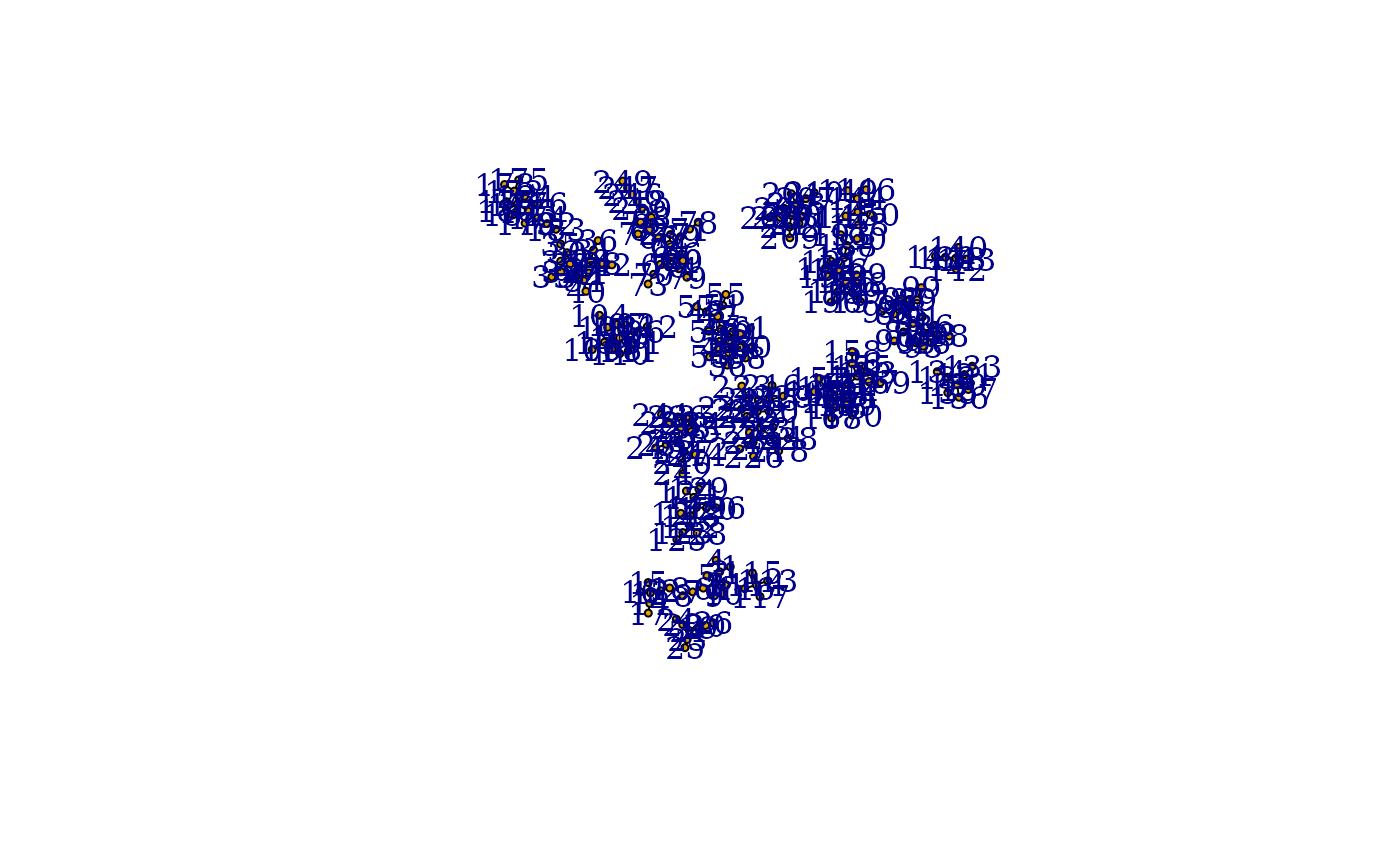Place several graphs on the same layout

## Usage

merge_coords(graphs, layouts, method = "dla")

layout_components(graph, layout = layout_with_kk, ...)

## Arguments

graphs

A list of graph objects.

layouts

A list of two-column matrices.

method

Character constant giving the method to use. Right now only dla is implemented.

graph

The input graph.

layout

A function object, the layout function to use.

...

Additional arguments to pass to the layout layout function.

## Value

A matrix with two columns and as many lines as the total number of vertices in the graphs.

## Details

merge_coords() takes a list of graphs and a list of coordinates and places the graphs in a common layout. The method to use is chosen via the method parameter, although right now only the dla method is implemented.

The dla method covers the graph with circles. Then it sorts the graphs based on the number of vertices first and places the largest graph at the center of the layout. Then the other graphs are placed in decreasing order via a DLA (diffision limited aggregation) algorithm: the graph is placed randomly on a circle far away from the center and a random walk is conducted until the graph walks into the larger graphs already placed or walks too far from the center of the layout.

The layout_components() function disassembles the graph first into maximal connected components and calls the supplied layout function for each component separately. Finally it merges the layouts via calling merge_coords().

plot.igraph(), tkplot(), layout(), disjoint_union()

Other graph layouts: add_layout_(), component_wise(), layout_as_bipartite(), layout_as_star(), layout_as_tree(), layout_in_circle(), layout_nicely(), layout_on_grid(), layout_on_sphere(), layout_randomly(), layout_with_dh(), layout_with_fr(), layout_with_gem(), layout_with_graphopt(), layout_with_kk(), layout_with_lgl(), layout_with_mds(), layout_with_sugiyama(), layout_(), norm_coords(), normalize()

## Author

Gabor Csardi csardi.gabor@gmail.com

## Examples


# create 20 scale-free graphs and place them in a common layout
graphs <- lapply(sample(5:20, 20, replace = TRUE),
barabasi.game,
directed = FALSE
)
layouts <- lapply(graphs, layout_with_kk)
lay <- merge_coords(graphs, layouts)
g <- disjoint_union(graphs)
plot(g, layout = lay, vertex.size = 3, labels = NA, edge.color = "black")